# Depth First Search Algorithm | DFS Example

## Depth First Search-

• Depth First Search or DFS is a graph traversal algorithm.
• It is used for traversing or searching a graph in a systematic fashion.
• DFS uses a strategy that searches “deeper” in the graph whenever possible.
• Stack data structure is used in the implementation of depth first search.

## DFS Example-

Consider the following graph-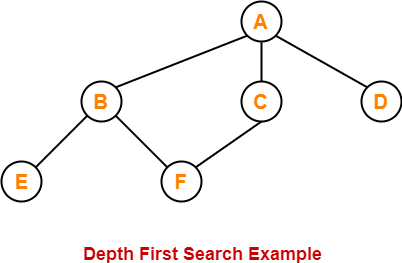The depth first search traversal order of the above graph is-

A, B, E, F, C, D

## Depth First Search Algorithm-

#### DFS (V,E)

for each vertex u in V[G]

do color[v] ← WHITE

π[v] ← NIL

time ← 0

for each vertex v in V[G]

do if color[v] ← WHITE

then Depth_First_Search(v)

### Depth_First_Search (v)

color[v] ← GRAY

time ← time + 1

d[v] ← time

for each vertex u adjacent to v

do if color[u] ← WHITE

π[u] ← v

Depth_First_Search(u)

color[v] ← BLACK

time ← time + 1

f[v] ← time

## Explanation-

The above depth first search algorithm is explained in the following steps-

## Step-01

Create and maintain 4 variables for each vertex of the graph.

For any vertex ‘v’ of the graph, these 4 variables are-

### 1. color[v]-

• This variable represents the color of the vertex ‘v’ at the given point of time.
• The possible values of this variable are- WHITE, GREY and BLACK.
• WHITE color of the vertex signifies that it has not been discovered yet.
• GREY color of the vertex signifies that it has been discovered and it is being processed.
• BLACK color of the vertex signifies that it has been completely processed.

### 2. Π[v]-

This variable represents the predecessor of vertex ‘v’.

### 3. d[v]-

This variable represents a timestamp when a vertex ‘v’ is discovered.

### 3. f[v]-

This variable represents a timestamp when the processing of vertex ‘v’ is completed.

## Step-02

For each vertex of the graph, initialize the variables as-

• color[v] = WHITE
• π[v] = NIL
• time = 0 (Global Variable acting as a timer)

### Step-03

Repeat the following procedure until all the vertices of the graph become BLACK-

Consider any white vertex ‘v’ and call the following Depth_First_Search function on it.

#### Depth_First_Search (G,v)

1. color[v] = GRAY

2. time = time + 1

3. d[v] = time

4. For each adjacent WHITE vertex ‘u’ of ‘v’, set π[u] = v and call Depth_First_Search (G,u)

5. color[v] = BLACK

6. time = time + 1

7. f[v] = time

## DFS Time Complexity-

The total running time for Depth First Search is θ (V+E).

## Types of Edges in DFS-

After a DFS traversal of any graph G, all its edges can be put in one of the following 4 classes-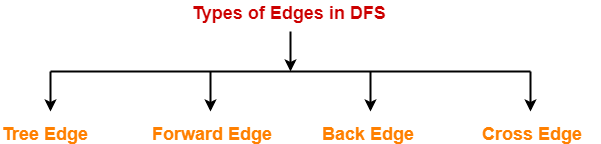1. Tree Edge
2. Back Edge
3. Forward Edge
4. Cross Edge

## 1. Tree Edge-

• A tree edge is an edge that is included in the DFS tree.

## 2. Back Edge-

 An edge from a vertex ‘u’ to one of its ancestors ‘v’ is called as a back edge. A self-loop is considered as a back edge.

A back edge is discovered when-

• DFS tries to extend the visit from a vertex ‘u’ to vertex ‘v’
• And vertex ‘v’ is found to be an ancestor of vertex ‘u’ and grey at that time.

### 3. Forward Edge-

 An edge from a vertex ‘u’ to one of its descendants ‘v’ is called as a forward edge.

A forward edge is discovered when-

• DFS tries to extend the visit from a vertex ‘u’ to a vertex ‘v’
• And finds that color(v) = BLACK and d(v) > d(u).

### 4. Cross Edge-

 An edge from a vertex ‘u’ to a vertex ‘v’ that is neither its ancestor nor its descendant is called as a cross edge.

A cross edge is discovered when-

• DFS tries to extend the visit from a vertex ‘u’ to a vertex ‘v’
• And finds that color(v) = BLACK and d(v) < d(u).

## Problem-

Compute the DFS tree for the graph given below-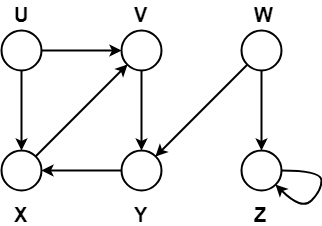Also, show the discovery and finishing time for each vertex and classify the edges.

## Solution-

Initially for all the vertices of the graph, we set the variables as-

• color[v] = WHITE
• π[v] = NIL
• time = 0 (Global)

Let us start processing the graph from vertex U.

### Step-01:

• color[U] = GREY
• time = 0 + 1 = 1
• d[U] = 1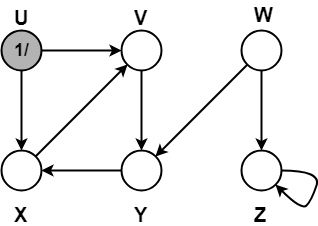### Step-02:

• π[V] = U
• color[V] = GREY
• time = 1 + 1 = 2
• d[V] = 2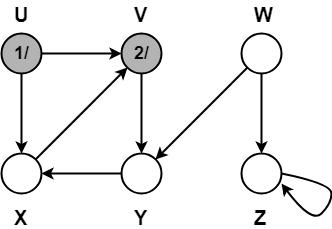### Step-03:

• π[Y] = V
• color[Y] = GREY
• time = 2 + 1 = 3
• d[Y] = 3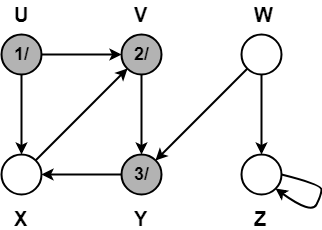### Step-04:

• π[X] = Y
• color[X] = GREY
• time = 3 + 1 = 4
• d[X] = 4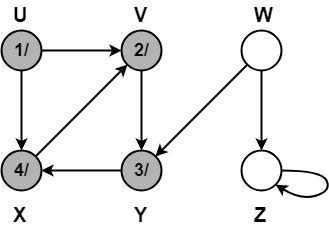### Step-05:

When DFS tries to extend the visit from vertex X to vertex V, it finds-

• Vertex V is an ancestor of vertex X since it has already been discovered
• Vertex V is GREY in color.

Thus, edge XV is a back edge.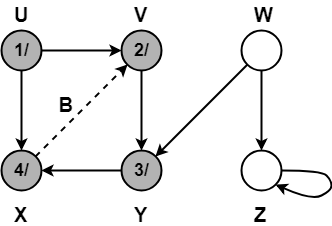### Step-06:

• color[X] = BLACK
• time = 4 + 1 = 5
• f[X] = 5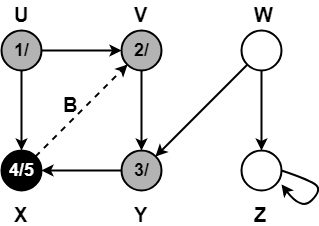### Step-07:

• color[Y] = BLACK
• time = 5 + 1 = 6
• f[Y] = 6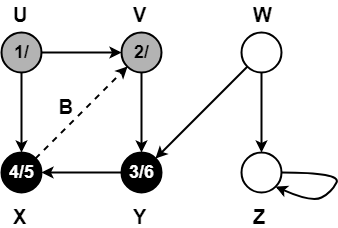### Step-08:

• color[V] = BLACK
• time = 6 + 1 = 7
• f[V] = 7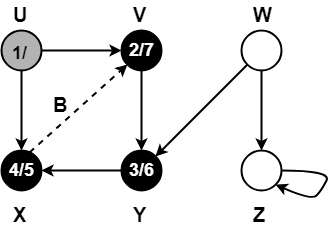### Step-09:

When DFS tries to extend the visit from vertex U to vertex X, it finds-

• Vertex X has already been completely processed i.e. vertex X has finished and is black.
• But vertex U has still not finished.

Alternatively,

When DFS tries to extend the visit from vertex U to vertex X, it finds-

• Color(X) = BLACK
• d(X) > d(U)

Thus, edge UX is a forward edge.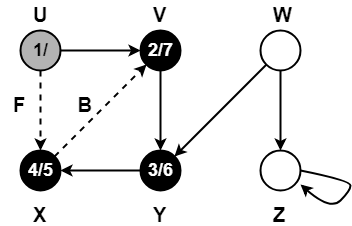### Step-10:

• color[U] = BLACK
• time = 7 + 1 = 8
• f[U] = 8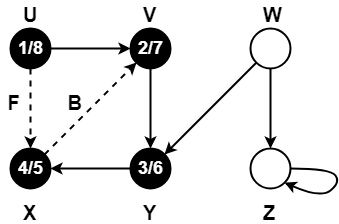### Step-11:

• color[W] = GREY
• time = 8 + 1 = 9
• d[W] = 9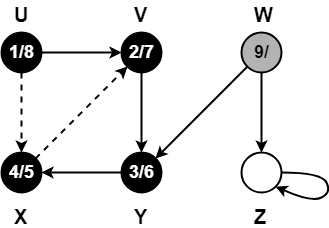### Step-12:

When DFS tries to extend the visit from vertex W to vertex Y, it finds-

• Vertex Y has already been completely processed i.e. vertex Y has finished.
• Vertex Y is neither a descendant nor an ancestor of vertex W.

Alternatively,

When DFS tries to extend the visit from vertex W to vertex Y, it finds-

• Color(Y) = BLACK
• d(Y) < d(W)

Thus, edge WY is a cross edge.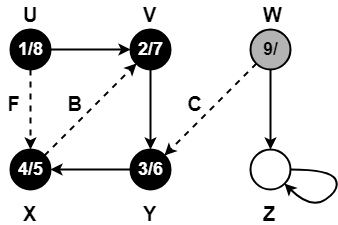### Step-13:

• π[Z] = W
• color[W] = GREY
• time = 9 + 1 = 10
• d[W] = 10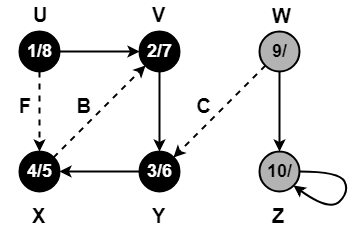### Step-14:

Since, self-loops are considered as back edges.

Therefore, self-loop present on vertex Z is considered as a back edge.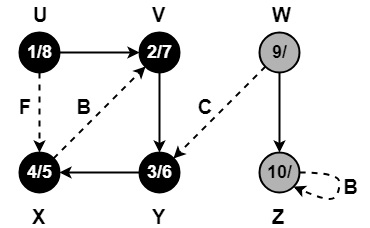### Step-15:

• color[Z] = BLACK
• time = 10 + 1 = 11
• f[Z] = 11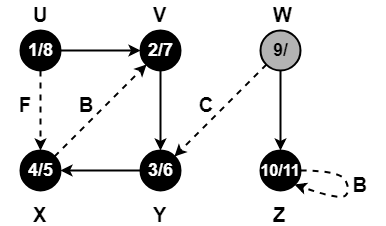### Step-16:

• color[W] = BLACK
• time = 11 + 1 = 12
• f[W] = 12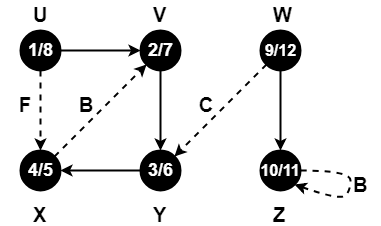Since all the vertices have turned black, so we stop.

This is how a given graph is traversed using Depth First Search (DFS) technique.

To gain better understanding about Depth First Search Algorithm,

Watch this Video Lecture

Get more notes and other study material of Design and Analysis of Algorithms.

Watch video lectures by visiting our YouTube channel LearnVidFun.

SummaryArticle Name
Depth First Search Algorithm | DFS Example
Description
Depth First Search Algorithm is a Graph Traversing Algorithm. DFS Algorithm is discussed Step by Step. DFS Example. DFS Algorithm searches deeper in the graph whenever possible.
Author
Publisher Name
Gate Vidyalay
Publisher Logo